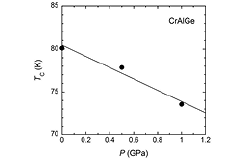ISSP - The institute for Solid State PhysicsFont Size: (S) / (M) / (L)

- Activity Report 2016 -

Y. Mitsui, Y. Uwatoko, and K. Koyama

Pressure Effect on Magnetic Properties of Weak Itinerant Electron Ferromagnet CrAlGeFig. 1. Isothermal M2 vs. H/M plots (Arrott plots) of CrAlGe under several pressures at 5 K. The solid lines are obtained by the least-squares calculation for the data.Fig. 2. Pressure dependence of TC. The solid line is least-squares fit.

CrAlGe with an orthorhombic TiSi2-type structure shows ferromagnetism below a Curie temperature TC of 80 K. The spontaneous magnetic moment ps and effective magnetic moment peff of CrAlGe were reported to be 0.41 μzB at 5 K and 1.89 μB, respectively . The paramagnetic moment pC was calculated to be 1.13 μB from peff by peff2 = pC (pC + 2), and the ratio pC/ps of CrAlGe was 2.8. Because the pC/ps is greater than unity, CrAlGe was classified as the weak itinerant electron ferromagnet (WIEF). The parameters of the energy scale of the spin fluctuation spectrum , T0 and TA were estimated to be 1.0 × 103 K and 4.0 × 103 K, respectively. However, the pressure effect on the magnetic properties of CrAlGe has not been clarified. In this study, we performed magnetization measurements of CrAlGe under high pressures up to 1 GPa.

The magnetization M measurements under hydrostatic pressure P up to 1.0 GPa were carried out using a superconducting quantum interference device magnetometer for 5 ≤ T ≤ 200 K and magnetic fields μ0H up to 5 T in a clamp-type piston cylinder pressure cell.

Figure 1 shows the isothermal M2 vs. H/M plots (Arrott plots) at 5 K under several P. ps at 0.1 MPa was determined to be 0.41 μB,/f.u. With increasing P, ps decreased, and dlnps/dP was –9.5 × 10-5 /MPa. Fig. 2 shows P dependence of TC. With increasing P, TC decreased, and dlnTC/dP was –8.2 × 10-5 /MPa. This is probably due to the increase of the 3d-bandwidth and the density of states in the vicinity of the Fermi level.

References

•  S. Yoshinaga, et al., Physics Procedia 75, 918 (2015).
•  Y. Takahashi, J. Phys.: Condens. Matter. 13, 6323 (2001).

Authers

• S. Yoshinagaa, Y. Mitsuia, R.Y. Umetsub, Y. Uwatoko, and K. Koyamaa
• aKagoshima University
• bTohoku University